Tamilnadu State Board New Syllabus Samacheer Kalvi 12th Maths Guide Pdf Chapter 11 Probability Distributions Ex 11.2 Textbook Questions and Answers, Notes.

## Tamilnadu Samacheer Kalvi 12th Maths Solutions Chapter 11 Probability Distributions Ex 11.2

Question 1.
Three fair coins are tossed simultaneously. Find the probability mass function for a number of heads that occurred.
Solution:
When three coins are tossed, the sample space is
S = {HHH, HHT, HTH, HTT, THH, THT, TTH, TTT}
‘X’ is the random variable that denotes the number of heads.
∴ ‘X’ can take the values of 0, 1, 2 and 3Sample space S = {HHH, HHT, HTH, THH, HTT, THT, TTH, TTT}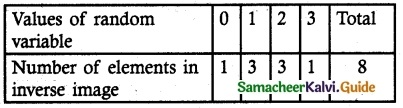Probability mass function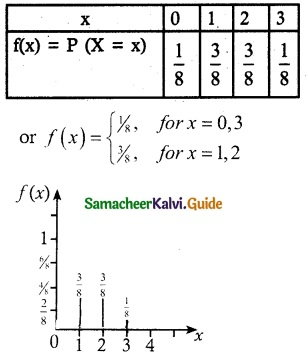Question 2.
A six sided die is marked ‘1’ on one face, ‘3’ on two of its faces and ‘5’ on remaining three faces. The die is thrown twice. If X denotes the total score in two throws, find
(i) the probability mass function
(ii) the cumulative distribution function
(iii) P(4 ≤ X < 10)
(iv) P(X ≥ 6)
Solution:
Let X be the random variable denotes the total score in two thrown of a die.
Sample space S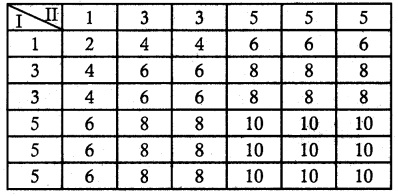n (S) = 36
X = {2, 4, 6, 8, 10}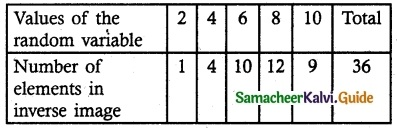(i) Probability mass function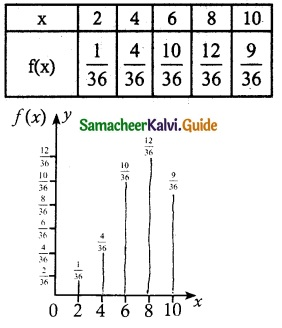(ii) Cumulative distribution function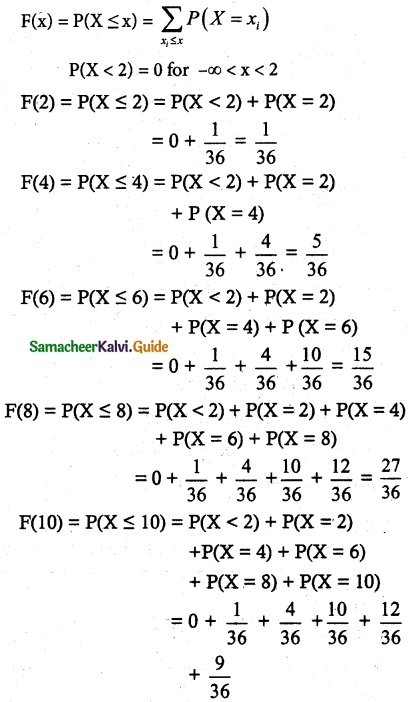= $$\frac { 36 }{ 36 }$$
= 1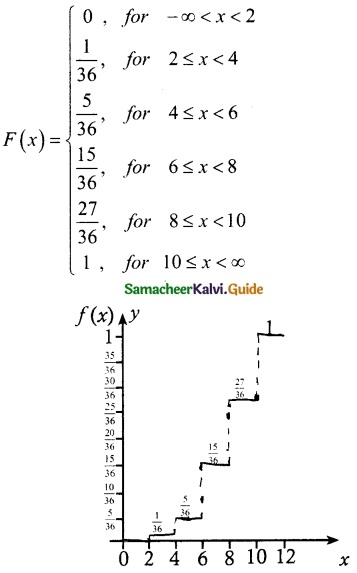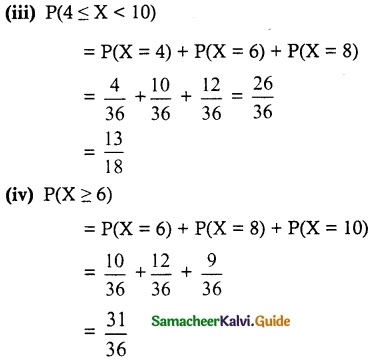Question 3.
Find the probability mass function and cumulative distribution function of a number of girl children in families with 4 children, assuming equal probabilities for boys and girls.
Solution:
Let X be the random variable denotes the number of girl child among 4 children
X = {0, 1, 2, 3, 4}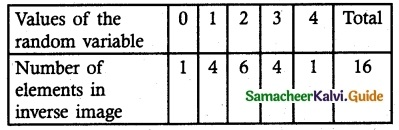(i) Probability mass function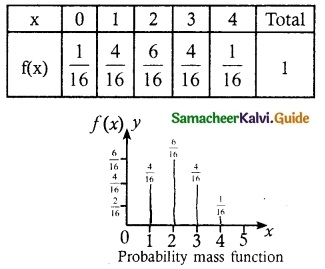(ii) Cumulative distribution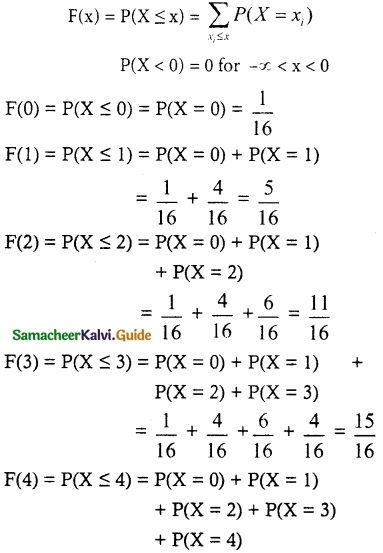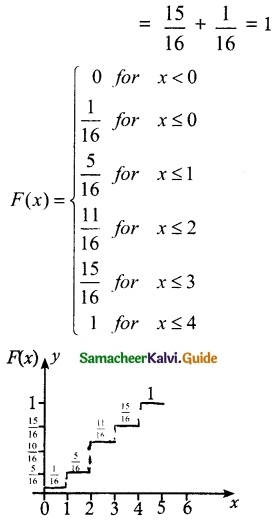Question 4.
Suppose a discrete random variable can only take the values 0, 1, and 2. The probability mass function is defined by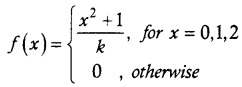Find (i) the value of k.
(ii) cumulative distribution function
(iii) P(X ≥ 1)
Solution: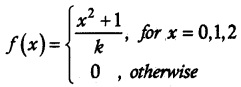(i) Given f is a probability mass function
$$\sum_{x}$$ f(x) = 1
Probability mass function is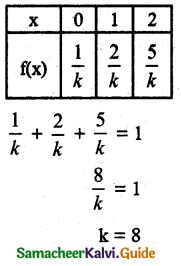(ii) Cumulative distribution function
F(0) = P(x ≤ 0)
= P(x = 0)
= $$\frac { 1 }{ 8 }$$
F(1) = P(x ≤ 1)
= P(x = 0) + P(x = 1)
= $$\frac { 1 }{ 8 }$$ + $$\frac { 2 }{ 8 }$$ = $$\frac { 3 }{ 8 }$$
F(2) = P(x ≤ 2)
= P(x = 0) + P(x = 1) + P(x = 2)
= $$\frac { 1 }{ 8 }$$ + $$\frac { 2 }{ 8 }$$ + $$\frac { 5 }{ 8 }$$ = 1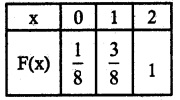(iii) P(X ≥ 1)
= P(X = 1) + P(X = 2)
= $$\frac { 2 }{ 8 }$$ + $$\frac { 5 }{ 8 }$$ + $$\frac { 7 }{ 8 }$$Question 5.
The cumulative distribution function of a discrete random variable is given by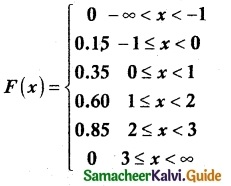Find
(i) the probability mass function
(ii) P(X < 1)
(iii) P(X ≥ 2)
Solution: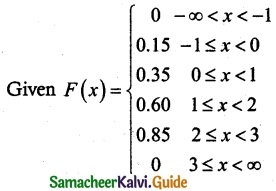f(-1) = P(X = -1) = F(-1) – F(-1) = 0.15 – 0 = 0.15
f(0) = P(X = 0) = F(0) – F(-1) = 0.35 – 0.15 = 0.20
f(1) = P(X = 1) = F(1) – F(0) = 0.60 – 0.35 = 0.25
f(2) = P(X = 2) = F(2) – F(1) = 0.85 – 0.60 = 0.25
f(3) = P(X = 3) = F(3) – F(2) = 1 – 0.85 = 0.15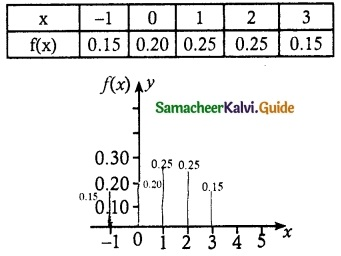(ii) P(X < 1)
P(X < 1) = P(X = -1) + P(X = 0)
= 0.15 + 0.20
= 0.35

(iii) P(X ≥ 2)
P(X ≥ 2) = P(X = 2) + P(X = 3)
= 0.25 + 0.15
= 0.40Question 6.
A random variable X has the following probability mass function.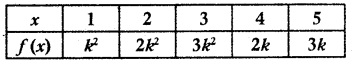Find
(i) the value of k
(ii) P(2 ≤ X < 5)
(iii) P(3 < X)
Solution:
(i) Given f(x) in a probability mass function
$$\sum_{x}$$ f(x) = 1
k² + 2k² + 3k² + 2k + 3k = 1
6k² + 5k = 1
6k² + 5k – 1 = 0
(k + 1) (6k – 1) = 0
k = $$\frac { 1 }{ 6 }$$
(k ≠ -1 neglecting negative terms)
Probability mass function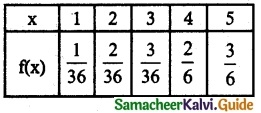(ii) P(2 ≤ X < 5)
P(2 ≤ X < 5) = P(X = 2) + P(X = 3) + P(X = 4)
= $$\frac { 2 }{ 36 }$$ + $$\frac { 3 }{ 36 }$$ + $$\frac { 2 }{ 6 }$$
= $$\frac { 2+3+12 }{ 36 }$$ + $$\frac { 17 }{ 36 }$$

(iii) P(X > 3) = P(X = 4) + P(X = 5)
= $$\frac { 2 }{ 6 }$$ + $$\frac { 3 }{ 6 }$$
= $$\frac { 5 }{ 6 }$$Question 7.
The cumulative distribution function of a discrete random variable is given by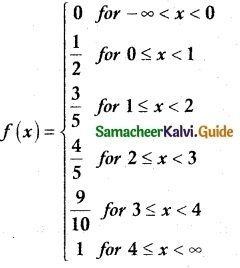Find
(i) the probability mass function
(ii) P(X < 3) and (iii) P(X ≥ 2)
Solution:
(i) Probability mass function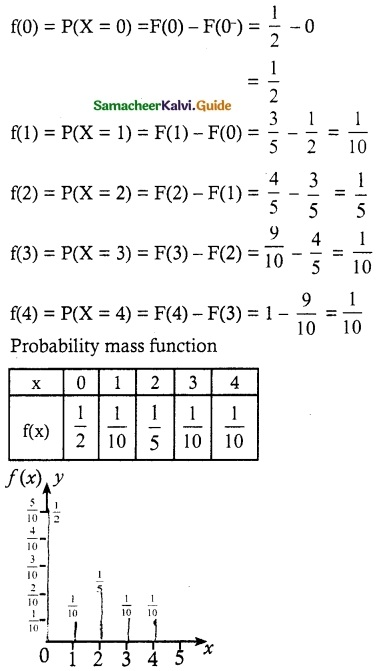(ii) P(X < 3)
P(X < 3) = P(X = 0) + P(X = 1) + P(X = 2)
= $$\frac { 1 }{ 2 }$$ + $$\frac { 1 }{ 10 }$$ + $$\frac { 1 }{ 5 }$$
= $$\frac { 4 }{ 5 }$$

(ii) P(X ≥ 2)
P(X ≥ 2) = P(X = 2) + P(X = 3) + P(X = 4)
= $$\frac { 1 }{ 5 }$$ + $$\frac { 1 }{ 10 }$$ + $$\frac { 1 }{ 10 }$$
= $$\frac { 4 }{ 10 }$$ = $$\frac { 2 }{ 5 }$$